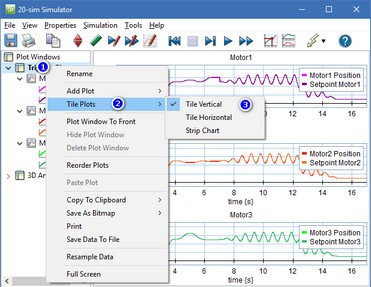﻿ 20-sim webhelp > Simulator > Running a Simulation > Arrange Plots

# Arrange Plots and Curves

A Plot Window can contain multiple plots with multiple curves. It can also contain a mix of curve plots and 3D animation plots.

## Change the plot window layout

By default, 20-sim will auto-arrange plots row-wise, then column-wise. It is possible to change the tile options to:

 • Tile Horizontal
 • Tile Vertical
 • Strip Chart

Strip Chart is a special version of Tile Vertical with a common X-axis for all plots.

To change the tile options, right-click on the plot window in the Plot Windows tree and select the menu Tile Plots.Change the plot tile options

## Reorder plots and curves

To change the order of plots within a plot window, you can drag-drop plots in the tree. It is also possible to move a plot from one plot window to another in this way.

Moving curves within plots and between plots is also possible via drag-drop.